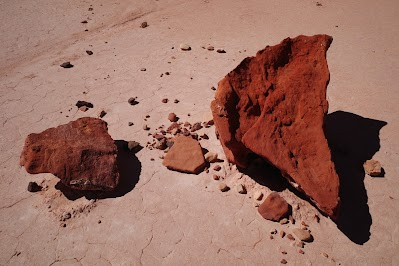### Introduction

If a small lump of a cohesive soil is desiccated, it can be observed that the volume decreases. A decrease in volume of a soil can only take place under the action of external compressive forces with a free drainage of the water contained in the pores. Both the above phenomena take place during the desiccation of a cohesive soil.

Owing the evaporation of water from the surface of the soil, the capillary menisci retreat into the narrowing pores exerting an increasing capillary pressure which is accompanied by increasing particle stresses and the reduction in the thickness of the adsorbed water films at the points of contact; this leads to a decrease in volume of the drying lump of soil.

The decrease in volume of a soil during desiccation is known as shrinkage. In the case of wetting of a desiccated soil a phenomenon opposite to the above described takes place, that is to say the soil swells. The water reaching the menisci on the surface of the soil decreases the capillary pressure which leads to the reduction of the particle contact stresses.

The adsorbed water films at the points of contact tend to achieve the complete hydration of the adsorbed actions by drawing water molecules back from the free water present in pores. The place of the molecules drawn into the adsorbed layers is taken up by the water drawn in from the surface of the soil. The volume of the soil increases, i.e. the soil swells. At the same time the water content of the soil increases while the pore water suction and the soil strength decrease.Clay soil

### The aim of the experiment

This experiment made to determine the free swell number, which gives an idea about the volume change of soils. This number uses in the classification of soils.

### Theory

The soil swells when its volume increases when the soil adsorbed water, the adsorbed water becomes at the points of contact of soil particle, so it is fill the spaces between the particles and increase the volume of the soils. The free swell number indicates the volume change of the soil, it can be calculated as follows:

Free swell number= [(Vf – Vi)/Vi]*100%

Where,

Vf= final volume of settled soil.

Vi= initial volume of soil sample.

The final volume takes directly by reading the volume from the cylinder.

The initial volume calculated as follows:

Ɣsolid= weight of solid(Ws) / volume of solid

Volume of solid (V initial)=weight of solid (Ws) /Ɣsolid

Where:

Ɣsolid=Ɣwater*S.G

### Instrument & materials

First, the instrument:

1. Two cylinders.
2. Balance.
3. Sieve #40.
4. Funnel.

Second, the materials:

Distilled water.

Two samples passing sieve #40 of oven dry soil from two different types.

### Procedure

1. Weight 10gm from each type of soils which passing sieve #40.
2. Fill each cylinder with distilled water up to 100ml mark.
3. Put first type of soil in the first cylinder, and the other type of soil in the other cylinder through the funnel.
4. After 24 hours take the readings of the volume of settled soil in each cylinder (final volume).

### Data & calculations

Sample 1 Sample 2
Weight of solid 10gm 10gm

First sample:

Ɣsolid= weight of solid(Ws) / volume of solid

Volume of solid (V initial) =weight of solid (Ws) /Ɣsolid

Ɣsolid=Ɣwater*S.G

Where,

specific gravity (S.G) =2.65

Unit weight of water (Ɣwater) = 1gm/cm^3

(V initial)=10/ (1*2.65)

=3.77cm^3

Free swell= [(Vf - V initial)/ V initial]*100%

= [(13.5 – 3.77)/3.77]*100%

=258.1%

Second sample:

Volume of solid (V initial) =weight of solid Ws /Ɣsolid

=10/ (1*2.65)

=3.77cm^3

Free swell= [(Vf - V initial)/ V initial]*100%

= [(19.5 – 3.77)/3.77]*100%

=417.1%

### Results & conclusion:

The free swell numbers obtained from the previous calculations are higher than 100%, which gives an idea that the soil is swelling when wetted by water, which means when these types of soils wetted with water their volumes increase in widely range.

1. In accurate in reading the balance.
2. Some soil particles pour out of the cylinder when put the soil in the cylinder, this gives reduction in weight of solid.
3. In accurate in reading the final volume from the cylinder.

When the soil swells the water content of the soil increases while the pore water suction and the soil strength decrease.